# Python | Pandas TimedeltaIndex.identical

• Last Updated : 28 Dec, 2018

Python is a great language for doing data analysis, primarily because of the fantastic ecosystem of data-centric python packages. Pandas is one of those packages and makes importing and analyzing data much easier.

Pandas` TimedeltaIndex.identical()` function determines if two Index objects contain the same elements. If the two objects under consideration contain same elements the function return True else the function return False.

Note : It is similar to equals, but check that other comparable attributes are also equal.

Syntax : TimedeltaIndex.identical(other)

Parameters :
other : other object

Return : boolean value

Example #1: Use `TimedeltaIndex.identical()` function to check if the elements contained in the two given TimedeltaIndex objects are identical or not.

 `# importing pandas as pd``import` `pandas as pd`` ` `# Create the first TimedeltaIndex object``tidx1 ``=` `pd.TimedeltaIndex(start ``=``'1 days 06:05:01.000030'``, periods ``=` `5``,``                                              ``freq ``=``'D'``, name ``=``'Koala'``)`` ` `# Create the second TimedeltaIndex object``tidx2 ``=` `pd.TimedeltaIndex(start ``=``'1 days 06:05:01.000030'``, periods ``=` `5``,``                                             ``freq ``=``'D'``, name ``=``'Koala'``)`` ` `# Print the first and second TimedeltaIndex object``print``(tidx1, ``'\n'``, tidx2)`

Output :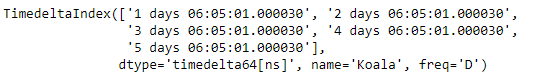Now we will use the `TimedeltaIndex.identical()` function to check if the values contained in tidx1 and tidx2 are identical or not.

 `# find if the elements are identical in tidx1 and tidx2``tidx1.identical(tidx2)`

Output :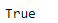As we can see in the output, the `TimedeltaIndex.identical()` function has returned `True` indicating that both the objects are identical to each other.

Example #2: Use `TimedeltaIndex.identical()` function to check if the elements contained in the two given TimedeltaIndex objects are identical or not.

 `# importing pandas as pd``import` `pandas as pd`` ` `# Create the TimedeltaIndex object``tidx1 ``=` `pd.TimedeltaIndex(data ``=``[``'1 days 02:00:00'``, ``'1 days 06:05:01.000030'``, ``                                 ``'1 days 02:00:00'``, ``'1 days 02:00:00'``,``                                           ``'21 days 06:15:01.000030'``])`` ` `# Create the second TimedeltaIndex object``tidx2 ``=` `pd.TimedeltaIndex(data ``=``[``'06:05:01.000030'``, ``'+23:59:59.999999'``, ``                          ``'22 day 2 min 3us 10ns'``, ``'+12:19:59.999999'``])`` ` `# Print the first and second TimedeltaIndex object``print``(tidx1, ``'\n'``, tidx2)`

Output :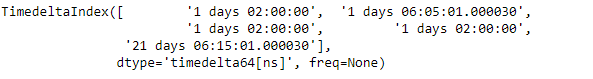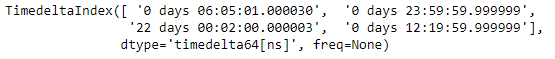Now we will use the `TimedeltaIndex.identical()` function to check if the values contained in tidx1 and tidx2 are same or not.

 `# find if the elements are identical in tidx1 and tidx2``tidx.identical(tidx2)`

Output :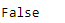As we can see in the output, the `TimedeltaIndex.identical()` function has returned `False` indicating that both the objects are not identical to each other.

My Personal Notes arrow_drop_up